Courses

# Test: Unit Cells, Lattices And Bragg's Equation

## 20 Questions MCQ Test Chemistry Class 12 | Test: Unit Cells, Lattices And Bragg's Equation

Description
This mock test of Test: Unit Cells, Lattices And Bragg's Equation for Class 12 helps you for every Class 12 entrance exam. This contains 20 Multiple Choice Questions for Class 12 Test: Unit Cells, Lattices And Bragg's Equation (mcq) to study with solutions a complete question bank. The solved questions answers in this Test: Unit Cells, Lattices And Bragg's Equation quiz give you a good mix of easy questions and tough questions. Class 12 students definitely take this Test: Unit Cells, Lattices And Bragg's Equation exercise for a better result in the exam. You can find other Test: Unit Cells, Lattices And Bragg's Equation extra questions, long questions & short questions for Class 12 on EduRev as well by searching above.
QUESTION: 1

### Only One Option Correct Type This section contains 9 multiple choice questions. Each question has four choices (a), (b), (c) and (d), out of which ONLY ONE is correct. Q. Bravais three-dimensional lattices are of

Solution:

14 types of three-dimensional Bravais lattices (primitive and centred unit cells

QUESTION: 2

### The lattice site in a pure crystal cannot be occupied by

Solution:

Lattice sites always contain atoms/molecules/ions. If atom is replaced by electron, then imperfection arises in the crystal and it would not be pure crystal

QUESTION: 3

### Number of formula units in unit cells of MgO (rock salt), ZnS (zinc blende) and Pt (fee) respectively are

Solution:

Ionic compounds are held by electrostatic forces of attraction between anions and cations.  These compounds form an extensive a 3 dimensional network where the boundaries between molecules are difficult to determine. The formula of ionic compounds are the empirical formula and are called the formula units.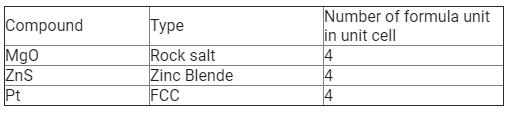QUESTION: 4

A compound is formed by elements A and B and has cubic structure. A-atoms are at the corners and B-atoms are at the face centres. Thus, it is

Solution: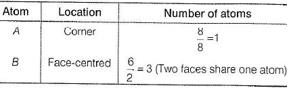QUESTION: 5

The mass of a unit cell of NaCL corresponds to

Solution: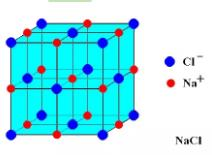The number of Na+ ions present in one unit cell = 12×1/4 + 1 =4 atoms per unit cell.

The number of Cl- ions present in one unit cell = 6×1/2 + 8×1/8 = 4 atoms per unit cell.

So, each unit cell has 4 atoms of each Na+ and Cl-. Hence there are total 4 molecules of NaCl in each unit cell.

Hence B

QUESTION: 6

At what angles for the first-order diffraction, spacing between two planes X respectively are λ and λ/2 ?

Solution:

(c) By Bragg's equation, spacing between two planes is given by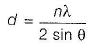For first-order diffraction, n =1

∴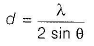If d = λ, then 2 sin θ =1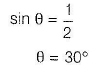if d = λ/2, then
sin θ = 1 => θ = 90o

QUESTION: 7

A solid has a structure in which W-atoms are located at the corners of a cubic lattice, OF-atoms at the centre of edges and Na atom at the centre of the cube. The formula for the compound is

Solution:

In a unit cell W atoms at the corner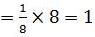O-atoms at the centre of edges =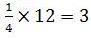Na-atoms at the centre of the cube = 1
W:O:Na = 1:3:1
Hence, formula is NaWO3

QUESTION: 8

The number of atoms in primitive cubic unit cell, body-centred cubic unit cell and face-centred cubic unit cell are A , B and C respectively. Select the correct values.

Solution:

(b) Number of atoms in the unit cell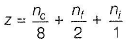where, nc = number of atoms at the corners nf = number of atoms at the faces ni = number of atoms inside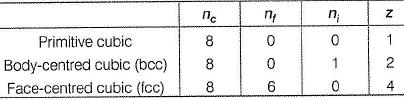QUESTION: 9

For a second order diffraction using X-ray B ragg’s equation, for a crystal with separation between two layers as d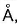, following graphical study was observed when varying 0 at different λ.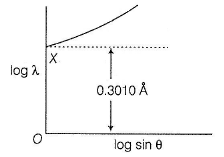What is the value of d?

Solution:

(c) By using Bragg’s equation,
nλ = 2d sin θ
where, n =2
(second order diffraction)
λ = d sin θ
or
log λ = log d + log sin θ
d is constant (distance between two planes) as θ changes, X also changes.
When θ = 90°, sin 90° =1, hence log sin 90°= 0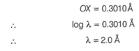*Multiple options can be correct
QUESTION: 10

One or More than One Options Correct Type
This section contains 3 multiple type questions. Each question has 4 choices (a), (b), (c) and (d), out of which ONE or MORE THAN ONE are correct.
In which of the following primitive cells axial distances or edge lengths (a, b, c)are different?

Solution:

(a,c,d )
(a) Orthorhombic : a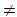bc
(b) Tetragonal: abc
(c) Mono clinic : abc
(d) Triclinic : abc

QUESTION: 11

Minimum interplanar spacing required for Bragg’s diffraction is:

Solution:

Explanation: Maximum value of incident angle can be 90° for which sine is 1. Hence d = λ/2 ( nλ = 2.d.sinθ)

QUESTION: 12

Which of the following Bravais lattices exist as face centered unit cell?

Solution:

Orthorhombic lattice exists as all four types of unit cells. Besides primitive cell, monoclinic lattice exists only as end centered cell while tetragonal exists only as body centered lattices.

QUESTION: 13

Comprehension Type
This section contains a passage describing theory, experiments, data, etc. Two questions related to the paragraph have been given. Each question has only one correct answer out of the given 4 options (a), (b), (c) and (d).
Perovskite (A), a mineral containing calcium (o), oxygen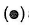and titanium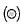crystallises in the following cubic unit cell: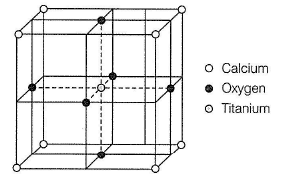Q.

What is the Formula of A?

Solution:

(b)
Calcium appears at eight corners, hence number of calcium atoms = 8/8 =1
Oxygen appears on six faces and two faces share one oxygen atom hence, number of oxygen atoms = 6/2 =3
Titanium appears at the centre, thus, number of Ti-atom = 1/1 =1
Thus, A is CaTi03.
Let the oxidation number of Ti = x
CaTi03
(+2) + x + 3 ( - 2 ) = 0
2 + x - 6 = 0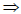x = + 4

QUESTION: 14

Perovskite (A), a mineral containing calcium (o), oxygenand titaniumcrystallises in the following cubic unit cell: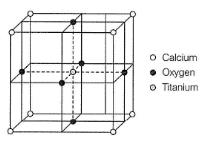Q. What is the oxidation number of titanium in the given lattice?

Solution:

(c)
Calcium appears at eight corners,hence number of calcium atoms = 8/8 =1
Oxygen appears on six faces and two faces share one oxygen atom hence, number of oxygen atoms = 6/2 =3
Titanium appears at the centre, thus, number of Ti-atom = 1/1 =1
Thus, A is CaTi03.
Let the oxidation number of Ti = x CaTi03
(+2) + x + 3 ( - 2 ) = 0
2 + x - 6 = 0x = + 4

QUESTION: 15

Matching List Type
Choices for the correct combination of elements from Column I and Column II are given as options (a), (b), (c) and (d), out of which one is correct.

Match the primitive unit cells given in Column I with their axial angles axial distances given in Column II and select the correct answer from the codes given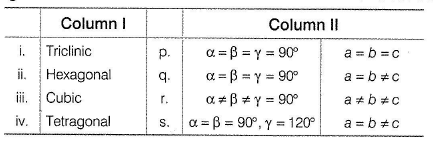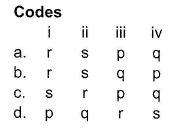Solution:

(i) Triclinic, abc and αβγ = 90° Thus, (i)→ (r)

(ii) Hexagonal, a = bc, α = β = 90°,γ = 120° Thus, (ii) → (s)

(iii) C u b ic ,a = b = c , α = β =γ = 90° Thus, (iii) → (p)

(iv) Tetragonal, a = bc, α = β =γ = 90

Thus, (iv) → (q)

*Answer can only contain numeric values
QUESTION: 16

One Integer Value Correct Type
This section contains 5 questions, when worked out will result in an integer value from 0 to 9 (both inclusive)
Q. A unit cell is characterised by how many parameters?

Solution:

(6) Edge length : a, b, c and angles between the edges :  α,β,γ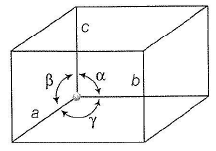*Answer can only contain numeric values
QUESTION: 17

How many types of primitive unit cells are there?

Solution:

Primitive unit cells are

(i) Cubic

(ii) Tetragonal

(iii) Orthorhombic

(iv) Hexagonal

(v) Rhombohedral

(vi) Monoclinic

(vii) Triclinic

QUESTION: 18

In which of the following Bravais lattices, not all axial angles are right angles?

Solution:

A rhombohedral is a prism whose base is shaped as a non-square rhombus. Hence two of its six faces are rhombuses while others are rectangles. This results in a geometry for which two of the axial angles are right angles but the third is not.

*Answer can only contain numeric values
QUESTION: 19

A second order Bragg’s diffraction of X-rays from a set of parallel planes separated by 1.155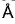occurs at an angle 60° using wavelength of x. What is the value of x?

Solution:

(1) By Bragg's equation,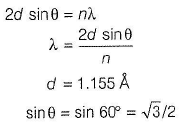n = 2 (second order diffraction)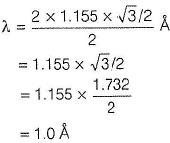*Answer can only contain numeric values
QUESTION: 20

Number of unit cells in 240 g of element X (atomic mass 40) which crystallises in bcc pattern in yN 0. What is the value of y?

Solution:

(3)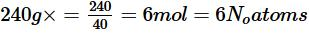Number of atoms in one unit cell of bcc structure =2
Thus, number of unit cells =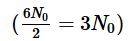Thus, y = 3